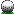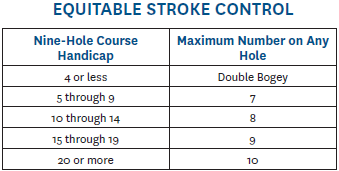HomeHandicap calculation The site uses the 2017 USGA Handicap formula to compute handicaps. The formula computes a handicap index which is converted to a handicap using the course slope. The maximum handicap index is 18.2 which converts to a maximum Village Greens front nine handicap of 19.5 Village Greens Woodridge  front-9 white: par: 36, slope: 121, rating: 35.2  back-9 white: par: 36, slope: 127, rating: 35.0  * you can find slopes for other tees in the USGA National Course DatabaseCourse Handicap is calculated as      Course Handicap = Handicap Index * (Slope of Tee on Course / 113)e.g. Player has handicap index of 6.0 and plays the back-9 white tees at Village Greens (slope: 127)      6.0 * (127/113) = 6.7 (rounded to nearest tenth)As of 4/1/2022 the method for computing 9-hole handicap indexes is as follows1. get the last 10 league round scores (these can include scores from previous seasons)2. For each score compute Handicap Differential (HC diff). The formula is    HC Diff = (AGS - course_rating) * 113) / course_slopewhere AGS is the Adjusted Gross Score. AGS can be lower than the actual round score, and is computed by adjusting the per hole score per round based on the following table for 9 holes3. sort the HC diffs in ascending order and use the lowest 6 if there more than 6, or use all if 6 or less (e.g. player has 5 HC Diffs so use all 5, another player has 10 HC Diffs so use the lowest 6).4. From the HC diffs compute the HC index using the formula    HC index = (average of HC diffs)*0.96 (with digits after tenths removed)        for example: ( (2.6 + 4.5 + 5.4 + 5.4 + 5.4 + 5.4) / 6 ) * 0.96 = 4.592 => 4.5 (with digits after tenths removed) 5. The handicap index is stored for the player and can be applied to any course using the formula     Course Handicap = HC Index * (Slope of Tee on Course / 113)     since the HC Index is for 9-holes you can double it to get an 18-hole HC Index for use when playing 18 holes.6. the maximum HC Index is 18.2 for men and 20.2 for womenNote this formula is not the official USGA formula but is based on the 2017 USGA formula with the following differences    - we use the last 10 scores (instead of 20 in the USGA formula).     - the maximum number of HC Diffs to use is 6 (instead of 10 in the USGA formula).     - we compute a HC index after the first round played where the USGA requires a minimum of 5 scores.